Home Practice
For learners and parents For teachers and schools
Textbooks
Full catalogue
Pricing SupportLog in

We think you are located in United States. Is this correct?

# Chapter 9: Acids and bases

## 9.1 Acids and bases (ESCNZ)

In this chapter learners will look at acids and bases. In Grade 11 learners were introduced to a lot of the concepts that are expanded on in this chapter. These include acid and base models and definitions, conjugate acid-base pairs, and some basic acid-base reactions. It is important that the learners have a good understanding of the work covered in Chapter 8, specifically equilibrium constants, before studying this chapter.

New concepts related to acids and bases will also be covered in this chapter. These include pH, more in depth information on titrations and indicators, and real world applications of acids and bases.

The following topics are covered in this chapter.

• What are acids and bases?

This section is largely revision from Grade 11. The Arrhenius and Brønsted-Lowry models for acids and bases are discussed. Conjugate acid-base pairs are also covered in detail in this section, including worked examples on how to determine the conjugate base of an acid and the conjugate acid of a base.

• Strong and weak acids and bases, and concentrated and dilute solutions

The concept of strong and weak acids and bases is often confused with the concept of concentrated and dilute solutions. It is important that the learners understand the difference between these concepts, which are discussed in detail in this section. The exercises will help to determine if the learners truly understand this work.

• $$\text{K}_{\text{a}}$$ and $$\text{K}_{\text{b}}$$

Although learners will not have encountered these terms before, the idea of an equilibrium constant should be familiar to them after encountering the equilibrium constant ($$\text{K}_{\text{c}}$$) in Chapter 8. The values of $$\text{K}_{\text{a}}$$ and $$\text{K}_{\text{b}}$$ should be tied to the ideas of strong and weak acids and bases.

• Acid-base reactions

These neutralisation reactions should be familiar to learners from Grade 11. They should revise these reactions through the revision activity provided. It is important that learners undersand how salts are formed from the acid and base, as well as the other products that are likely in each type of reaction.

• pH

The pH scale is a logarithmic scale with a base of $$\text{10}$$. So a change in pH of $$\text{3}$$ is a significant change in the concentration of $$\text{H}^{+}$$ ions. A good understanding of pH is also essential to understanding how indicators work. This section covers why a neutralised solution will not necessarily have a pH of $$\text{7}$$, the concept of $$\text{K}_{\text{w}}$$, and the hydrolysis of salts. Indicators are also covered in this section and it important that learners understand how to choose an indicator for an experiment before starting the next section on titrations.

• Titrations

Titrations are covered in great detail in this section. This includes learners preparing their own standard solutions, performing several titration experiments, and doing titration calculations. The learners will be working with acids and bases a lot in this section and so should be reminded of proper laboratory safety procedures. Each experiment will take a large amount of time and at least an entire class should be dedicated to these experiments. If possible one class should be for the preparation of a standard solution and the first titration experiment, while another, separate class is for to the second titration experiment.

• Applications of acids and bases

The applications covered are the production of chlorine in industry and the use of bases in hair products. The production of chlorine is not covered in great detail in this chapter, for more detail see Chapter 13. The hair care products discussed include: hair permanent waving applications, hair relaxers, and hair dyes. The learners must also perform an experiment using drain cleaners or caustic soda. These products have a high pH and can burn skin. This lesson can also be used to consolidate information by going through the chapter summary and doing the end of chapter exercises.

There are several experiments in this chapter. The learners will be using dangerous chemicals and should be properly instructed on the correct use of safety equipment, including safety goggles, gloves and protective clothing. They should also be reminded not to sniff any chemicals as the fumes can be dangerous as well. More information on laboratory procedures as well as safety precautions is provided in Chapter 1 (Science skills).

In the informal assessment the learners must determine the temperature change caused by the exothermic reaction of sodium hydroxide and hydrochloric acid. They should understand why the temperature stops changing when all the sodium hydroxide has been neutralised.

The formal assessment requires that the learners prepare a standard solution of sodium hydroxide. It is important that the learners understand why the mass must be known to a high accuracy, why the beakers must be rinsed with water (with the rinsings added to the volumetric flask), why the sides of the volumetric flask must be washed with water, and why it is important to have their eye level with the mark on the volumetric flask when filling to that mark.

### What are acids and bases? (ESCP2)

We encounter many examples of acids and bases in our daily lives. Some common examples of household items that contain acids are vinegar (contains acetic acid), lemon juice (contains citric and ascorbic acid), wine (contains tartaric acid). These acids are often found to have a sour taste. Hydrochloric acid, sulfuric acid and nitric acid are examples of acids that are more likely to be found in laboratories and industry.

Hydrochloric acid is also found in the gastric juices in the stomach. Fizzy drinks contain carbonic acid, while tea and wine contain tannic acid. People even use acids in an artistic process known as acid etching. In acid etching, a metal is covered in a waxy material that is resistant to acid. The bare metal is then exposed in the desired pattern and the sample is placed in an acid bath. The top layers of the exposed metal are permanently removed, creating the desired image. Some examples of this are shown below (Figure 9.2).

Bases that you may know about include sodium hydroxide (commonly known as caustic soda), ammonium hydroxide and ammonia. Some of these are found in household cleaning products. Bases are usually found to have a bitter taste and feel slippery (soap is a good example). Acids and bases are also important commercial components in the fertiliser, plastics, and petroleum refining industries. Some common acids and bases, and their chemical formulae, are shown in Table 9.1.

 Acid Formula Base Formula Hydrochloric acid $$\text{HCl}$$ Sodium hydroxide $$\text{NaOH}$$ Sulfuric acid $$\text{H}_{2}\text{SO}_{4}$$ Potassium hydroxide $$\text{KOH}$$ Nitric acid $$\text{HNO}_{3}$$ Magnesium hydroxide $$\text{Mg}(\text{OH})_{2}$$ Oxalic acid $$\text{H}_{2}\text{C}_{2}\text{O}_{4}$$ Calcium hydroxide $$\text{Ca}(\text{OH})_{2}$$ Sulfurous acid $$\text{H}_{2}\text{SO}_{3}$$ Sodium bicarbonate $$\text{NaHCO}_{3}$$ Phosphoric acid $$\text{H}_{3}\text{PO}_{4}$$ Sodium carbonate $$\text{Na}_{2}\text{CO}_{3}$$ Acetic (ethanoic) acid $$\text{CH}_{3}\text{COOH}$$ Ammonium hydroxide $$\text{NH}_{4}\text{OH}$$ Carbonic acid $$\text{H}_{2}\text{CO}_{3}$$ Ammonia $$\text{NH}_{3}$$

Table 9.1: Some common acids and bases and their chemical formulae.

Dissociation is the breaking apart of a molecule to form smaller molecules or ions, usually in a reversible manner.

The wild lupin plant takes nitrogen from the atmosphere and creates ammonia. It uses that ammonia to fertilise the soil for itself and the surrounding plants.Sodium bicarbonate is used in baking. It reacts with the acids in dough to release carbon dioxide. The carbon dioxide then causes the dough to expand.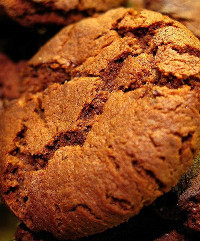## Naturally occurring acids and bases

Do research on three naturally occurring acids, and one naturally occurring base. That is, acids and bases that are found mostly in plants (not man-made).

• Where the acid or base is found (e.g. the plant in which it occurs).

• Indigenous uses of the plant.

• What the chemical composition of the acid or base is.

The next section deals with some models used to describe acids and bases. It is important to have a definition so that acids and bases can be correctly identified in reactions.

### Models for acids and bases (ESCP3)

For the acids you will encounter this year, an acid is a molecule that donates a $$\text{H}^{+}$$ ion.

Substances that will act as a base include hydroxides, oxides, carbonates or hydrogen carbonate, among others. Bases often release free hydroxide ions ($$\text{OH}^{-}$$) when dissociating in water.

#### Arrhenius model for acids and bases

A number of models for acids and bases have been developed over the years. One of the earliest was the Arrhenius definition. In 1884, Arrhenius discovered that water dissociates (splits up) into hydronium $$(\text{H}_{3}\text{O}^{+})$$ and hydroxide $$(\text{OH}^{-})$$ ions according to the following equation:

$$2\text{H}_{2}\text{O}(\text{ℓ})$$ $$\rightleftharpoons {\color{red}{\text{H}_{3}{\text{O}}^{+}{\text{(aq)}}}} + {\color{blue}{\text{OH}^{-}{\text{(aq)}}}}$$

Another way of writing this is:

$$\text{H}_{2}\text{O}(\text{ℓ})$$ $$\rightleftharpoons {\color{red}{\text{H}^{+}{\text{(aq)}}}} + {\color{blue}{\text{OH}^{-}{\text{(aq)}}}}$$

Arrhenius described an acid as a compound that forms $$\text{H}_{3}\text{O}^{+}$$ when added to water. An Arrhenius acid therefore increases the concentration of $$\text{H}_{3}\text{O}^{+}$$ ions ( [$$\text{H}_{3}\text{O}^{+}$$] ) in water. Arrhenius described a base as a compound that dissociates in water to form $$\text{OH}^{-}$$ ions. An Arrhenius base therefore increases the concentration of $$\text{OH}^{-}$$ ions ([$$\text{OH}^{-}$$] ) in water.

Arrhenius acids and bases

An Arrhenius acid forms $$\text{H}_{3}\text{O}^{+}$$ in water (increases [$$\text{H}_{3}\text{O}^{+}$$]). An Arrhenius base forms $$\text{OH}^{-}$$ in water (increases [$$\text{OH}^{-}$$]).

 $$\color{red}{\textbf{Arrhenius acid}}$$ $$\text{increases }[\color{red}{\text{H}_{3}\text{O}^{+}}]$$ $$\color{blue}{\textbf{Arrhenius base}}$$ $$\text{increases }[\color{blue}{\text{OH}^{-}}]$$

Table 9.2: The Arrhenius definition of acids and bases.

Look at the following examples showing the dissociation of hydrochloric acid and sodium hydroxide:

1. $${\color{red}{\text{HCl(aq)}}}$$ + $$\text{H}_{2}\text{O}(\text{ℓ})$$ $$\to {\color{red}{\text{H}_{3}{\text{O}}^{+}{\text{(aq)}}}}$$ + $$\text{Cl}^{-}(\text{aq})$$

$${\color{red}{\text{Hydrochloric acid}}}$$ in water increases the concentration of $${\color{red}{\text{H}_{3}{\text{O}}^{+}}}$$ ions and is therefore an $${\color{red}{\textit{acid}}}$$.

2. $${\color{blue}{\text{NaOH(s)}}}$$ + $$\text{H}_{2}\text{O}(\text{ℓ})$$ $$\to$$ $$\text{Na}^{+}(\text{aq})$$ + $${\color{blue}{\text{OH}^{-}{\text{(aq)}}}}$$ + $$\text{H}_{2}\text{O}(\text{ℓ})$$

$${\color{blue}{\text{Sodium hydroxide}}}$$ in water increases the concentration of $${\color{blue}{\text{OH}^{-}}}$$ ions and is therefore a $${\color{blue}{\textit{base}}}$$.

However, this definition can only be used for acids and bases in water. Since there are many reactions which do not occur in water, it was important to come up with a much broader definition for acids and bases.

#### Brønsted-Lowry model for acids and bases

In 1923, Lowry and Brønsted took the work of Arrhenius further to develop a broader definition for acids and bases. The Brønsted-Lowry model defines acids and bases in terms of their ability to donate or accept protons (Table 9.3).

Hydrogen atoms contain only one proton. $$\text{H}^{+}$$ is a hydrogen atom that has lost its electron and is often called a proton.

Brønsted-Lowry acids and bases

An acid is a substance that donates (gives away) protons ($$\text{H}^{+}$$). A base is a substance that accepts (takes) protons.

Under the Brønsted-Lowry definition: an $$\color{red}{\text{acid}}$$ is a $$\color{red}{\textbf{proton donor}}$$; a $$\color{blue}{\text{base}}$$ is a $$\color{blue}{\textbf{proton}}$$ $$\color{blue}{\textbf{acceptor}}$$.

 $$\color{red}{\textbf{Brønsted-Lowry acid}}$$ $$\text{donates H}^{+}$$ $$\text{proton }\color{red}{\textbf{donor}}$$ $$\color{blue}{\textbf{Brønsted-Lowry base}}$$ $$\text{accepts H}^{+}$$ $$\text{proton }\color{blue}{\textbf{acceptor}}$$

Table 9.3: The Brønsted-Lowry definition of acids and bases.

Below are some examples:

1. $$\text{HCl}(\text{aq}) + \text{NH}_{3}(\text{g})$$ $$\to$$ $$\text{NH}_{4}^{+}(\text{aq}) + \text{Cl}^{-}(\text{aq})$$

In order to decide which substance is a $${\color{red}{\text{proton donor}}}$$ and which is a $${\color{blue}{\text{proton acceptor}}}$$, we need to look at what happens to each reactant. The reaction can be broken down as follows:

$$\text{HCl}(\text{aq})$$ $$\to$$ $${\color{darkgreen}{\text{H}^{+}}}$$(aq) + $$\text{Cl}^{-}(\text{aq})$$

• $$\text{HCl}$$ donates a $$\color{darkgreen}{\text{proton}}$$.

It is a $${\color{red}{\textit{proton donor}}}$$ and is therefore the $${\color{red}{\textbf{acid}}}$$.

$$\text{NH}_{3}(\text{g})$$ + $${\color{darkgreen}{\text{H}^{+}}}{\text{(aq)}} \to$$ $$\text{NH}_{4}^{+}(\text{aq})$$

• $$\text{NH}_{3}$$ accepts a $$\color{darkgreen}{\text{proton}}$$.

It is a $$\color{blue}{\textit{proton acceptor}}$$ and is therefore the $$\color{blue}{\textbf{base}}$$.

2. $$\text{CH}_{3}\text{COOH}(\text{aq}) + \text{H}_{2}\text{O}(\text{ℓ})$$ $$\to$$ $$\text{H}_{3}\text{O}^{+}(\text{aq}) + \text{CH}_{3}\text{COO}^{-}(\text{aq})$$

The reaction can be broken down as follows:

$$\text{CH}_{3}\text{COOH}(\text{aq})$$ $$\to$$ $$\text{CH}_{3}\text{COO}^{-}(\text{aq})$$ + $$\color{darkgreen}{\text{H}^{+}}$$(aq)

• $$\text{CH}_{3}\text{COOH}$$ donates a $$\color{darkgreen}{\text{proton}}$$.

It is a $$\color{red}{\textit{proton donor}}$$ and is therefore the $$\color{red}{\textbf{acid}}$$.

$$\text{H}_{2}\text{O}(\text{ℓ})$$ + $$\color{darkgreen}{\text{H}^{+}}\text{(aq)} \to$$ $$\text{H}_{3}\text{O}^{+}(\text{aq})$$

• Water accepts a $$\color{darkgreen}{\text{proton}}$$.

It is a $$\color{blue}{\textit{proton acceptor}}$$ and is therefore the $$\color{blue}{\textbf{base}}$$.

3. $$\text{NH}_{3}(\text{g}) + \text{H}_{2}\text{O}(\text{ℓ})$$ $$\to$$ $$\text{NH}_{4}^{+}(\text{aq}) + \text{OH}^{-}(\text{aq})$$

The reaction can be broken down as follows:

$$\text{H}_{2}\text{O}(\text{ℓ})$$ $$\to$$ $$\text{OH}^{-}(\text{aq})$$ + $$\color{darkgreen}{\text{H}^{+}}$$(aq)

• Water donates a $$\color{darkgreen}{\text{proton}}$$.

It is a $$\color{red}{\textit{proton donor}}$$ and is therefore the $$\color{red}{\textbf{acid}}$$.

$$\text{NH}_{3}(\text{g})$$ + $$\color{darkgreen}{\text{H}^{+}}\text{(aq)} \to$$ $$\text{NH}_{4}^{+}(\text{aq})$$

• Ammonia accepts a $$\color{darkgreen}{\text{proton}}$$.

It is a $${\color{blue}{\textit{proton acceptor}}}$$ and is therefore the $${\color{blue}{\textbf{base}}}$$.

Notice that in example $$\text{2}$$ $$\color{darkgreen}{\textbf{water}}$$ acted as a $${\color{blue}{\textbf{base}}}$$, while in example $$\text{3}$$ $$\color{darkgreen}{\textbf{water}}$$ acted as an $${\color{red}{\textbf{acid}}}$$. Water can act as both an acid and a base depending on the reaction. A substance that can act as either an acid or a base is called amphoteric.

Amphoteric

An amphoteric substance is one that can act as an acid in one reaction, or a base in another reaction.

An amphoteric substance that contains both acidic and basic functional groups is called an ampholyte.

An amphiprotic substance is an amphoteric substance that can donate a proton in one reaction (a Brønsted-Lowry acid), or accept a proton in another reaction (a Brønsted-Lowry base).

Amphiprotic

An amphiprotic substance can donate a proton in one reaction, or accept a proton in another reaction.

Substances such as ammonia ($$\text{NH}_{3}$$), zinc oxide ($$\text{ZnO}$$), and beryllium hydroxide ($$\text{Be}(\text{OH})_{2}$$) are amphoteric. Water and ammonia are also amphiprotic.

An acid that releases only one $${\color{darkgreen}{\text{H}^{+}}}$$ ion per molecule of acid (e.g. $$\color{darkgreen}{\text{H}}$$Cl) is referred to as monoprotic. An acid that can release two $${\color{darkgreen}{\text{H}^{+}}}$$ ions per molecule of acid (e.g. $$\color{darkgreen}{\text{H}_{2}}$$$$\text{SO}_{4}$$) is referred to as diprotic. Any acid than can donate more than one $${\color{darkgreen}{\text{H}^{+}}}$$ ion per molecule of acid can be referred to as polyprotic (this means that diprotic acids are also polyprotic).

### Conjugate acid-base pairs (ESCP4)

Look at the reaction between hydrochloric acid and ammonia to form ammonium and chloride ions:

$$\text{HCl}(\text{aq}) + \text{NH}_{3}(\text{g})$$ $$\rightleftharpoons$$ $$\text{NH}_{4}^{+}(\text{aq}) + \text{Cl}^{-}(\text{aq})$$

Looking at the forward reaction (i.e. the reaction that proceeds from left to right), the changes that take place can be shown as follows:

• $${\color{red}{\text{HCl(aq)}}} \to {\text{Cl}}^{-}{\text{(aq)}} + {\color{darkgreen}{{\text{H}}^{+}{\text{(aq)}}}}$$

• $${\color{blue}{\text{NH}_{3}{\text{(g)}}}} + {\color{darkgreen}{\text{H}^{+}{\text{(aq)}}}} \to {\text{NH}_{4}^{+}{\text{(aq)}}}$$

• In the forward reaction, HCl is a proton donor ($${\color{red}{\textbf{acid}}}$$) and $$\text{NH}_{3}$$ is a proton acceptor ($${\color{blue}{\textbf{base}}}$$).

Looking at the reverse reaction (i.e. the reaction that proceeds from right to left), the changes that take place are as follows:

• $${\color{blue}{\text{Cl}^{-}{\text{(aq)}}}} + {\color{darkgreen}{{\text{H}}^{+}{\text{(aq)}}}} \to {\text{HCl(aq)}}$$

• $${\color{red}{\text{NH}_{4}^{+}{\text{(aq)}}}} \to {\text{NH}_{3}{\text{(g)}}} + {\color{darkgreen}{{\text{H}}^{+}{\text{(aq)}}}}$$

• In the reverse reaction, the chloride ion ($$\text{Cl}^{-}$$) is the proton acceptor ($${\color{blue}{\textbf{base}}}$$) and the ammonium ion ($$\text{NH}_{4}^{+}$$) is the proton donor ($${\color{red}{\textbf{acid}}}$$).

In the forward reaction $$\color{red}{\text{HCl}}$$ donates a proton ($$\text{H}^{+}$$) to form $$\color{blue}{\text{Cl}^{-}}$$. In the reverse reaction $$\color{blue}{\text{Cl}^{-}}$$ accepts a proton to form $$\color{red}{\text{HCl}}$$. $$\color{blue}{\text{Cl}^{-}}$$ is the $$\color{blue}{\text{conjugate base}}$$ of the $$\color{red}{\text{acid HCl}}$$. So $$\text{HCl}$$ and $$\text{Cl}^{-}$$ are a $${\color{orange}{\textbf{conjugate acid-base pair}}}$$.

Similarly, in the forward reaction $$\color{blue}{\text{NH}_{3}}$$ accepts a proton ($$\text{H}^{+}$$) to form $$\color{red}{{\text{NH}}_{4}^{+}}$$. In the reverse reaction $$\color{red}{{\text{NH}}_{4}^{+}}$$ donates a proton to form $$\color{blue}{\text{NH}_{3}}$$. $$\color{red}{\text{NH}_{4}^{+}}$$ is the $$\color{red}{\text{conjugate acid}}$$ of the $$\color{blue}{\text{base NH}_{3}}$$. So $$\text{NH}_{3}$$ and $$\text{NH}_{4}^{+}$$ are a $${\color{purple}{\textbf{conjugate acid-base pair}}}$$.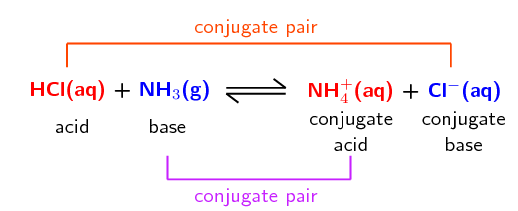The reaction between ammonia and water is another example: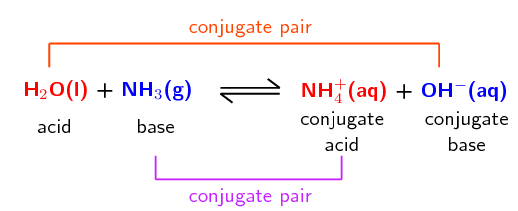Conjugate acid-base pair

A conjugate acid-base pair contains two compounds that differ only by a hydrogen ion ($$\text{H}^{+}$$) and a charge of $$\text{+1}$$.

## Worked example 1: Conjugate acid-base pairs

Determine the conjugate acid-base pairs for the following reaction:

$$\text{HNO}_{3}(\text{aq}) + \text{OH}^{-}(\text{aq})$$ $$\rightleftharpoons$$ $$\text{NO}_{3}^{-}(\text{aq}) + \text{H}_{2}\text{O}(\text{ℓ})$$

### Which reactant is an acid and which is a base?

$$\text{HNO}_{3}$$ is nitric acid. It donates a proton in the forward reaction:

$$\text{HNO}_{3}(\text{aq})$$ $$\to$$ $$\text{NO}_{3}^{-}(\text{aq}) + \text{H}^{+}(\text{aq})$$

$$\text{OH}^{-}$$ accepts a proton in the forward reaction and is therefore the base:

$$\text{OH}^{-}(\text{aq}) + \text{H}^{+}(\text{aq})$$ $$\to$$ $$\text{H}_{2}\text{O}(\text{ℓ})$$

### Which product is the conjugate base of the acid?

Nitric acid ($$\text{HNO}_{3}$$) donates a proton to become $$\text{NO}_{3}^{-}$$. In the reverse reaction $$\text{NO}_{3}^{-}$$ accepts a proton to become $$\text{HNO}_{3}$$. Therefore $$\text{NO}_{3}^{-}$$ is the conjugate base of $$\text{HNO}_{3}$$.

$$\text{NO}_{3}^{-}(\text{aq}) + \text{H}^{+}(\text{aq})$$ $$\to$$ $$\text{HNO}_{3}(\text{aq})$$

### Which product is the conjugate acid of the base?

$$\text{OH}^{-}$$ accepts a proton to become $$\text{H}_{2}\text{O}$$. In the reverse reaction $$\text{H}_{2}\text{O}$$ donates a proton to become $$\text{OH}^{-}$$. Therefore $$\text{H}_{2}\text{O}$$ is the conjugate acid of $$\text{OH}^{-}$$.

$$\text{H}_{2}\text{O}(\text{ℓ})$$ $$\to$$ $$\text{OH}^{-}(\text{aq}) + \text{H}^{+}(\text{aq})$$

### Label the conjugate acid-base pairs in this reaction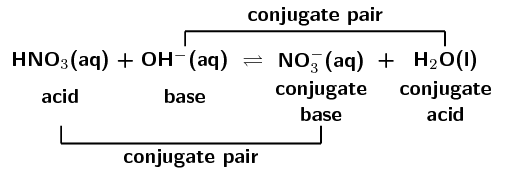temp text

## Acids and bases

Textbook Exercise 9.1

In each of the following reactions, label the conjugate acid-base pairs.

$$\text{H}_{2}\text{SO}_{4}(\text{aq}) + \text{H}_{2}\text{O}(\text{aq})$$ $$\rightleftharpoons$$ $$\text{H}_{3}\text{O}^{+}(\text{aq}) + \text{HSO}_{4}^{-}(\text{aq})$$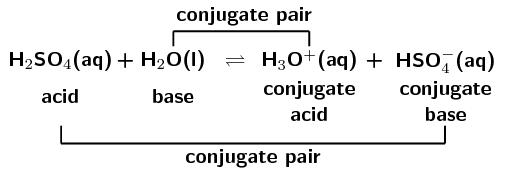$$\text{NH}_{4}^{+}(\text{aq}) + \text{F}^{-}(\text{aq})$$ $$\rightleftharpoons$$ $$\text{HF}(\text{aq}) + \text{NH}_{3}(\text{g})$$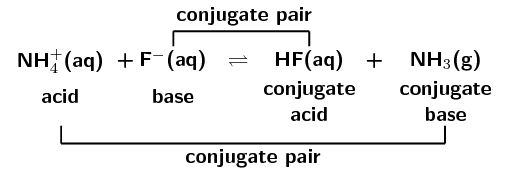$$\text{H}_{2}\text{O}(\text{ℓ}) + \text{CH}_{3}\text{COO}^{-}(\text{aq})$$ $$\rightleftharpoons$$ $$\text{OH}^{-}(\text{aq}) + \text{CH}_{3}\text{COOH}(\text{aq})$$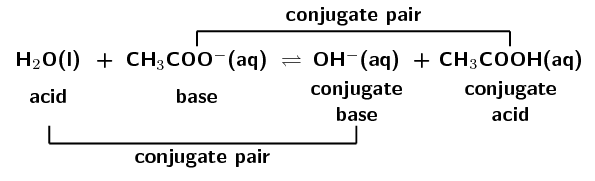$$\text{H}_{2}\text{SO}_{4}(\text{aq}) + \text{Cl}^{-}(\text{aq})$$ $$\rightleftharpoons$$ $$\text{HCl}(\text{aq}) + \text{HSO}_{4}^{-}(\text{aq})$$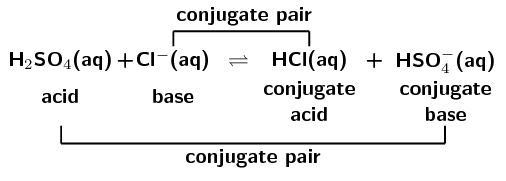Write the conjugate base for:

$$\text{H}_{2}\text{CO}_{3}$$

This is an acid. A proton needs to be donated to form the conjugate base:

$$\text{H}_{2}\text{CO}_{3}$$ $$\to$$ $$\text{HCO}_{3}^{-} + \text{H}^{+}$$

$$\text{HCO}_{3}^{-}$$ is the conjugate base of $$\text{H}_{2}\text{CO}_{3}$$.

$$\text{H}_{3}\text{O}^{+}$$

This is an acid. A proton needs to be donated to form the conjugate base:

$$\text{H}_{3}\text{O}^{+}$$ $$\to$$ $$\text{H}_{2}\text{O} + \text{H}^{+}$$

$$\text{H}_{2}\text{O}$$ is the conjugate base of $$\text{H}_{3}\text{O}^{+}$$.

$$\text{H}_{2}\text{PO}_{4}^{-}$$

This is an acid. A proton needs to be donated to form the conjugate base:

$$\text{H}_{2}\text{PO}_{4}^{-}$$ $$\to$$ $$\text{HPO}_{4}^{2-} + \text{H}^{+}$$

$$\text{HPO}_{4}^{2-}$$ is the conjugate base of $$\text{H}_{2}\text{PO}_{4}^{-}$$.

Write the conjugate acid for:

$$\text{OH}^{-}$$

This is a base. A proton needs to be accepted to form the conjugate acid:

$$\text{OH}^{-} + \text{H}^{+}$$ $$\to$$ $$\text{H}_{2}\text{O}$$

$$\text{H}_{2}\text{O}$$ is the conjugate acid of $$\text{OH}^{-}$$.

$$\text{CN}^{-}$$

This is a base. A proton needs to be accepted to form the conjugate acid:

$$\text{CN}^{-} + \text{H}^{+}$$ $$\to$$ $$\text{HCN}$$

$$\text{HCN}$$ is the conjugate acid of $$\text{CN}^{-}$$.

$$\text{CO}_{3}^{2-}$$

This is a base. A proton needs to be accepted to form the conjugate acid:

$$\text{CO}_{3}^{2-} + \text{H}^{+}$$ $$\to$$ $$\text{HCO}_{3}^{-}$$

$$\text{HCO}_{3}^{-}$$ is the conjugate acid of $$\text{CO}_{3}^{2-}$$.

### Strong and weak acids and bases (ESCP5)

The term dissociate should be used for ionic solids that break into their component parts when dissolved in water. Ionise should be used when a reaction with water takes place and ions, that were not present in the original compound, are formed.

For example, $$\text{NaOH}$$ is an ionic solid composed of $$\text{Na}^{+}$$ ions and $$\text{OH}^{-}$$ ions. Upon dissolution it dissociates into its component parts.

On the other hand, $$\text{HCl}$$ is a covalent molecule. When dissolved in water it ionises to form $$\text{H}^{+}$$ and $$\text{Cl}^{-}$$ ions.

In general, strong bases dissociate, bases such as ammonia and organic amines ionise. All acids ionise.

#### Strong acids and bases

A strong acid or base is one that will almost completely dissociate or ionise to form ions in solution. That is, a large percentage of the moles of a strong acid or base will form ions when added to water.

Strong acid and strong base
A strong acid or base is one that almost completely dissociates to form ions in solution.

$$\text{HCl}$$ is a strong acid. For example if $$\text{100 000}$$ molecules of $$\text{HCl}$$ are added to water and $$\text{90 000}$$ ionise to form $$\text{H}^{+}$$ and $$\text{Cl}^{-}$$ ions, then there is a large amount of ionisation. This is what makes $$\text{HCl}$$ a strong acid.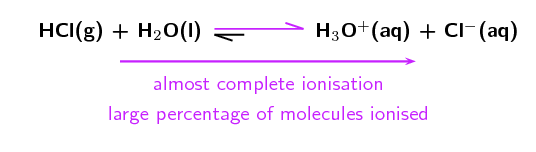The unequal double arrows in the reaction equation indicate that the equilibrium position favours the formation of ions.

There are three strong acids that we commonly find: $$\text{HCl}$$ (hydrochloric acid), $$\text{HNO}_{3}$$ (nitric acid) and $$\text{H}_{2}\text{SO}_{4}$$ (sulfuric acid). Two strong bases that are commonly found are: $$\text{NaOH}$$ (sodium hydroxide) and $$\text{KOH}$$ (potassium hydroxide).

#### Weak acids and bases

A weak acid or base is one where only a small percentage of molecules will dissociate to form ions in solution.

Weak acid and weak base
A weak acid or base is one where only a small percentage of molecules dissociate to form ions in solution.

$$\text{HF}$$ is a weak acid. For example if $$\text{100 000}$$ molecules of HF are added to water and only $$\text{100}$$ ionise to form $$\text{H}^{+}$$ and $$\text{F}^{-}$$ ions, then there is only a small amount of ionisation. This is what makes $$\text{HF}$$ a weak acid.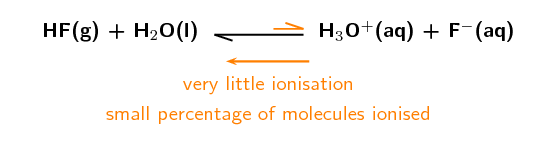The unequal double arrows in the reaction equation indicate that the equilibrium position does not favour the formation of ions.

An example of a weak base is $$\text{Mg}(\text{OH})_{2}$$ which will only dissociate partially into $$\text{Mg}^{2+}$$ and $$\text{OH}^{-}$$ ions.

### Dilute and concentrated solutions (ESCP6)

A different concept to strong and weak is the concept of concentrated and dilute. Where $$\color{purple}{\text{strong and weak}}$$ refer to the $$\color{purple}{\text{characteristic of a compound}}$$, $$\color{orange}{\text{concentrated and dilute}}$$ refer to the $$\color{orange}{\text{characteristic of a solution}}$$. Thus a strong acid can be prepared as either a concentrated or a dilute solution. A solution of which the exact concentration is known is called a standard solution.

Standard solution

A standard solution is one where the exact concentration of solute in a solvent is known.

#### Concentrated solutions

A concentrated solution is one where a large amount of a substance (solute) has been added to a solvent. Note that both strong and weak acids and bases can be used in concentrated solutions.

Concentrated solution
A concentrated solution is one where there is a high ratio of dissolved substance (e.g. acid or base) to solvent.

#### Dilute solutions

A dilute solution is one where a small amount of a substance has been added to a solvent. Note that both strong and weak acids and bases can be used in dilute solutions.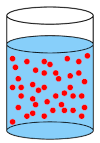A concentrated solution has a lot of solute molecules (red circles) in the solvent.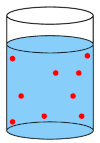A dilute solution has few solute molecules (red circles) in the solvent.

Dilute solution
A dilute solution is one where there is a low ratio of dissolved substance to solvent.

A concentrated solution can be made from a strong or a weak acid or base. A dilute solution can also be made from a strong or a weak acid or base. Whether a solution is concentrated or dilute depends on how much of the acid or base was added to the solvent.

A strong base that is also concentrated would be a base that almost completely dissociates when added to a solution, and you also add a large amount of the base to the solution.

A weak acid that is also dilute would be an acid where only a small percentage of molecules ionise when added to a solution, and you also add only a small amount of the acid to the solution. Table 9.4 summarises these concepts.

The electrical conductivity of a solution depends on the concentration of mobile ions in the solution. This means that a concentrated solution of a strong acid or base will have a high electrical conductivity, while a dilute solution of a weak acid or base will have a low electrical conductivity.

Electric current is the movement of charged particles. Therefore, the more ions (charged particles) there are in a solution, the greater the electric current that can be conducted through the solution by the charged particles. This is the electrical conductivity of a solution.

Mobile ions are ions that are able to move. These include ions in solution and ions in melted ionic materials. The ions in ionic solids are not mobile.

 $$\color{red}{\textbf{Acid}}$$ $$\color{blue}{\textbf{Base}}$$ Strong high percentage forms ions in solution high percentage forms ions in solution Weak only a small percentage forms ions in solution only a small percentage forms ions in solution Concentrated large number of moles of $$\color{red}{\text{acid}}$$ in solution large number of moles of $$\color{blue}{\text{base}}$$ in solution Dilute small number of moles of $$\color{red}{\text{acid}}$$ in solution small number of moles of $$\color{blue}{\text{base}}$$ in solution

Table 9.4: A summary of the properties of strong, weak, concentrated, and dilute acids and bases.

## Worked example 2: Acids and bases

Solution 1 contains $$\text{100}$$ $$\text{dm^{3}}$$ of $$\text{HCl}$$ added to $$\text{10}$$ $$\text{dm^{3}}$$ of water. Almost all the $$\text{HCl}$$ molecules ionise in the solution.

Solution 2 contains $$\text{0,01}$$ $$\text{g}$$ of $$\text{Mg}(\text{OH})_{2}$$ added to $$\text{1 000}$$ $$\text{dm^{3}}$$ of water. Only a small percentage of the $$\text{Mg}(\text{OH})_{2}$$ molecules dissociate in the solution.

Say whether these solutions:

1. Contain a strong or weak acid or base.

2. Are concentrated or dilute.

### Are the compounds acids or bases?

$$\text{HCl}$$ is hydrochloric acid. It would donate a proton and is an acid. $$\text{Mg}(\text{OH})_{2}$$ is magnesium hydroxide and is a base.

### What makes an acid or base strong or weak?

Almost complete ionisation or dissociation means an acid or base is strong. Only a small amount of ionisation or dissociation means an acid or base is weak.

### Are the compounds strong or weak acids and bases?

Almost all the $$\text{HCl}$$ molecules ionise in the solution, therefore $$\text{HCl}$$ is a strong acid.

Only a small percentage of the $$\text{Mg}(\text{OH})_{2}$$ molecules dissociate, therefore $$\text{Mg}(\text{OH})_{2}$$ is a weak base.

### What makes a solution concentrated or dilute?

A concentrated solution has a high ratio of solute to solvent. A dilute solution has a low ratio of solute to solvent.

### Are the solutions concentrated or dilute?

$$\text{100}$$ $$\text{dm^{3}}$$ of $$\text{HCl}$$ is added to $$\text{10}$$ $$\text{dm^{3}}$$ of water. This is a high ratio, therefore the solution of $$\text{HCl}$$ is concentrated.

$$\text{0,01}$$ $$\text{g}$$ of $$\text{Mg}(\text{OH})_{2}$$ is added to $$\text{1 000}$$ $$\text{dm^{3}}$$ of water. This is a low ratio, therefore the solution of $$\text{Mg}(\text{OH})_{2}$$ is dilute.

Solution 1 is a concentrated solution of a strong acid.

Solution 2 is a dilute solution of a weak base.

## Worked example 3: Acids and bases

Solution 1 contains $$\text{0,01}$$ $$\text{dm^{3}}$$ of $$\text{NaOH}$$ added to $$\text{800}$$ $$\text{dm^{3}}$$ of water. Almost all the $$\text{NaOH}$$ molecules dissociate in the solution.

Solution 2 contains $$\text{100}$$ $$\text{g}$$ of $$\text{HF}$$ added to $$\text{10}$$ $$\text{dm^{3}}$$ of water. Only a small percentage of the $$\text{HF}$$ molecules ionise in the solution.

Say whether these solutions:

1. Contain a strong or weak acid or base.

2. Are concentrated or dilute.

### Are the compounds acids or bases?

$$\text{NaOH}$$ is sodium hydroxide and is a base. $$\text{HF}$$ is hydrofluoric acid and is an acid.

### What makes an acid or base strong or weak?

Almost complete ionisation or dissociation means an acid or base is strong. Only a small amount of ionisation or dissociation means an acid or base is weak.

### Are the compounds strong or weak acids and bases?

Almost all the $$\text{NaOH}$$ molecules dissociate in the solution, therefore $$\text{NaOH}$$ is a strong base.

Only a small percentage of the $$\text{HF}$$ molecules ionise, therefore $$\text{HF}$$ is a weak acid.

### What makes a solution concentrated or dilute?

A concentrated solution has a high ratio of solute to solvent. A dilute solution has a low ratio of solute to solvent.

### Are the solutions concentrated or dilute?

$$\text{0,01}$$ $$\text{g}$$ of $$\text{NaOH}$$ is added to $$\text{800}$$ $$\text{dm^{3}}$$ of water. This is a low ratio, therefore the solution of $$\text{NaOH}$$ is dilute.

$$\text{100}$$ $$\text{dm^{3}}$$ of $$\text{HF}$$ is added to $$\text{10}$$ $$\text{dm^{3}}$$ of water. This is a high ratio, therefore the solution of $$\text{HF}$$ is concentrated.

Solution 1 is a dilute solution of a strong base.

Solution 2 is a concentrated solution of a weak acid.

To calculate the concentration of a solution we use the formula:

$\text{c (mol·dm}^{-3}{\text{)}}=\dfrac{\text{n (mol)}}{\text{V (dm}^{3}{\text{)}}}$

## Worked example 4: Calculating concentration

$$\text{0,27}$$ $$\text{g}$$ of $$\text{H}_{2}\text{SO}_{4}$$ is added to $$\text{183,7}$$ $$\text{dm^{3}}$$ of water. Calculate the concentration of the solution.

### List the information you have and the information you need

$$V = \text{183,7}$$ $$\text{dm^{3}}$$; $$m = \text{0,27}$$ $$\text{g}$$

The volume ($$V$$) and the mass ($$m$$) are given. The number of moles ($$n$$) needs to be calculated. To do that the molar mass ($$M$$) needs to be calculated.

### Make sure all given units are correct and convert them if necessary

All the units are correct.

### What equations will be necessary to calculate the concentration?

$$c\text{ (mol·dm}^{-3}{\text{)}}=\dfrac{n\text{ (mol)}}{ V\text{ (dm}^{3}{\text{)}}}$$

$$n\text{ (mol)} = \dfrac{m\text{ (g)}}{ M\text{(g·mol}^{-1}{\text{)}}}$$

### Calculate the number of moles of the acid in the solution

\begin{align*} M(\text{H}_{2}\text{SO}_{4}) &= (\text{2} \times \text{1,01}) + \text{32,1} + (\text{4} \times \text{16}) \\ & = \text{98,12} \text{ g·mol$^{-1}$} \end{align*}

\begin{align*} n &= \dfrac{m}{M} \\ &= \dfrac{\text{0,27} {\text{ g}}}{\text{98,12} {\text{ g·mol}}^{-1}} \\ &= \text{0,00275}\ldots \text{ mol} \end{align*}

### Calculate the concentration of the solution

\begin{align*} c &= \dfrac{n}{V} \\ &= \dfrac{\text{0,00275}\ldots {\text{ mol}}}{\text{183,7} {\text{ dm}}^{3}} \\ &= \text{0,00001497} \ldots \text{mol·dm$^{-3}$} \\ & = \text{1,50} \times \text{10}^{-\text{5}} \text{ mol·dm$^{-3}$} \end{align*}

## Worked example 5: Calculating concentration

$$\text{16,4}$$ $$\text{g}$$ of $$\text{KOH}$$ is added to $$\text{12,9}$$ $$\text{cm^{3}}$$ of water. Calculate the concentration of the solution.

### List the information you have and the information you need

$$V = \text{12,9}$$ $$\text{cm^{3}}$$; $$m = \text{16,4}$$ $$\text{g}$$

The volume ($$V$$) and the mass ($$m$$) are given. The number of moles ($$n$$) needs to be calculated. To do that the molar mass ($$M$$) needs to be calculated.

### Make sure all given units are correct or convert them

The volume needs to be converted to $$\text{dm^{3}}$$.

$$V = \text{12,9}$$ $$\text{cm^{3}}$$ $$\times \dfrac{\text{0,001} {\text{ dm}}^{3}}{\text{1} {\text{ cm}}^{3}} = \text{0,0129}$$ $$\text{dm^{3}}$$

### What equations will be necessary to calculate the concentration?

$$c\text{ (mol·dm}^{-3}{\text{)}}=\dfrac{n\text{ (mol)}}{ V\text{ (dm}^{3}{\text{)}}}$$

$$n\text{ (mol)} = \dfrac{m\text{ (g)}}{ M\text{ (g·mol}^{-1}{\text{)}}}$$

### Calculate the number of moles of base in the solution

$$M(\text{KOH}) = \text{39,1} + \text{16} + \text{1,01} = \text{56,11} \text{ g·mol^{-1}}$$.

$$n = \dfrac{m}{M} = \dfrac{\text{16,4} { \text{ g}}}{\text{56,11} {\text{ g·mol}}^{-1}} = \text{0,292} \text{ mol}$$

### Calculate the concentration of the solution

$$c =\dfrac{n}{V} = \dfrac{\text{0,292} {\text{ mol}}}{\text{0,0129} {\text{ dm}}^{3}} = \text{22,64}$$ $$\text{mol·dm^{-3}}$$

temp text

## Types of acids and bases

Textbook Exercise 9.2

Say whether the solutions of the acids and bases in the following situations are concentrated or dilute.

For every $$\text{1}$$ mole of a solvent there are $$\text{50}$$ moles of lithium hydroxide ($$\text{LiOH}$$).

Concentrated. There are many more moles of the base than the solvent.

For every $$\text{100}$$ moles of a solvent there are $$\text{5}$$ moles of nitric acid ($$\text{HNO}_{3}$$).

Dilute. There are many more moles of the solvent than the acid.

95% of an unknown acid donates protons when the acid is added to water. The pH of the final solution is $$\text{6,5}$$.

Is the acid a strong or a weak acid? Give a reason for your answer.

The acid is a strong acid. 95% of the acid ionises in the water. This means that it almost completely forms ions in solution and is therefore a strong acid.

Is a solution with a pH of $$\text{6,5}$$ strongly or weakly acidic?

The solution is weakly acidic.

Is the solution concentrated or dilute? Give a reason for your answer.

The solution is dilute. Although this is a strong acid the solution is only weakly acidic. This means that there must be a very small number of moles of the acid in the total volume of the solution.

Calculate the concentration for of the following solutions.

$$\text{27}$$ $$\text{g}$$ of sodium bicarbonate ($$\text{NaHCO}_{3}$$) added to $$\text{22,6}$$ $$\text{cm^{3}}$$ of water.

You have the volume ($$V$$) and the mass ($$m$$). You need the number of moles ($$n$$) and the molar mass ($$M$$).

$$M(\text{NaHCO}_{3}) = \text{23} + \text{1,01} + \text{12} + (\text{3} \times \text{16}) = \text{84,01} \text{ g·mol^{-1}}$$.

$$n(\text{NaHCO}_{3}) = \dfrac{\text{m(g)}} {\text{M (g·mol}^{-1}{\text{)}}} = \dfrac{\text{27} {\text{ g}}}{\text{84,01} {\text{ g·mol}}^{-1}} = \text{0,32} \text{ mol}$$

$$V = \text{22,6} \text{cm^{3}} \times \dfrac{\text{0,001} {\text{ dm}}^{3}}{\text{1} {\text{ cm}}^{3}} = \text{0,0226} \text{ dm^{3}}$$

$$c(\text{NaHCO}_{3}) = \dfrac{ \text{n (mol)}}{\text{V (dm}^{3}{\text{)}}} = \dfrac{\text{0,32} {\text{ mol}}}{\text{0,0226} {\text{ dm}}^{3}} = \text{14,16} \text{ mol·dm^{-3}}$$

$$\text{0,893}$$ $$\text{mol}$$ of phosphoric acid ($$\text{H}_{3}\text{PO}_{4}$$) added to $$\text{4,79}$$ $$\text{dm^{3}}$$ of a solvent.

You have the volume ($$V$$) and number of moles ($$n$$). You can calculate the concentration.

$$c(\text{H}_{3}\text{PO}_{4}) = \dfrac{ \text{n (mol)}}{\text{V (dm}^{3}{\text{)}}} = \dfrac{\text{0,893} {\text{ mol}}}{\text{4,79} {\text{ dm}}^{3}} = \text{0,186} \text{ mol·dm^{-3}}$$

$$\text{32,8}$$ $$\text{mg}$$ of hydrochloric acid ($$\text{HCl}$$) added to $$\text{12,76}$$ $$\text{cm^{3}}$$ of water.

You have the volume ($$V$$) and the mass ($$m$$). You need the number of moles ($$n$$) and the molar mass ($$M$$).

$$M(\text{HCl}) = \text{1,01} + \text{35,45} = \text{36,46} \text{ g·mol^{-1}}$$.

$$m(\text{HCl}) = \text{32,8} \text{mg} \times \dfrac{\text{0,001} { \text{ g}}}{\text{1} {\text{ mg}}} = \text{0,0328} \text{ g}$$

$$n(\text{HCl}) = \dfrac{\text{m (g)}}{ \text{M (g·mol}^{-1}{\text{)}}} = \dfrac{\text{0,0328} {\text{ g}}}{\text{36,46} {\text{ g·mol}}^{-1}} = \text{9,0} \times \text{10}^{-\text{4}} \text{ mol}$$

$$V = \text{12,76} \text{cm^{3}} \times \dfrac{\text{0,001} {\text{ dm}}^{3}}{\text{1} {\text{ cm}}^{3}} = \text{0,01276} \text{ dm^{3}}$$

$$c(\text{HCl}) = \dfrac{\text{n (mol)}}{ \text{V (dm}^{3}{\text{)}}} = \dfrac{\text{9,0} \times \text{10}^{-\text{4}} {\text{ mol}}}{\text{0,01276} {\text{ dm}}^{3}} = \text{0,07} \text{ mol·dm^{-3}}$$

$$\text{1,12}$$ $$\text{dm^{3}}$$ of a $$\text{6,54}$$ $$\text{mol·dm^{-3}}$$ concentration solution of ammonia ($$\text{NH}_{3}$$) added to $$\text{0,50}$$ $$\text{dm^{3}}$$ of water.

You have the volume (V) and original concentration of the ammonia solution, you need number of moles (n) in that original solution.

$$\text{c} = \dfrac{\text{n (mol)}}{ \text{V (dm}^{3}{\text{)}}}$$. Therefore $$n = c \times V$$

$$n$$(original solution of $$\text{NH}_{3}$$) $$= \text{6,54} \text{mol·dm^{-3}} \times \text{1,12} \text{dm^{3}} = \text{7,32} \text{mol}$$.

The final volume used to calculate the concentration must be the volume of the water plus the volume of ammonia added.

$$V$$(total) = $$\text{0,50}$$ $$\text{dm^{3}} + \text{1,12} \text{dm^{3}} = \text{1,62} \text{ dm^{3}}$$

$$c$$(final solution of $$\text{NH}_{3}$$) = $$\dfrac{n\text{ (mol)}}{ V\text{ (dm}^{3}{\text{)}}} = \dfrac{\text{7,32} {\text{ mol}}}{\text{1,62} {\text{ dm}}^{3}}$$ = $$\text{4,52}$$ $$\text{mol·dm^{-3}}$$

### $$K_{a}$$ and $$K_{b}$$ (ESCP7)

The equilibrium constant for the ionisation process of an acid (the extent to which ions are formed in solution) is given by the term $${\text{K}_{\color{red}{\textbf{a}}}}$$, while that for a base is given by $${\text{K}_{\color{blue}{\textbf{b}}}}$$. These equilibrium constants are a way of determining whether the acid or base is weak or strong.

Remember from Chapter 8 that we calculate $$\text{K}$$ as follows:

$${\text{K}} = \dfrac{\text{[products]}}{\text{[reactants]}}$$

#### Acids

• Strong acids

Consider the ionisation of $$\text{HBr}$$:

$$\color{red}{\text{HBr(g)}}$$ + $$\text{H}_{2}\text{O}(\text{ℓ})$$ $$\to$$ $$\text{H}_{3}\text{O}^{+}(\text{aq}) + \text{Br}^{-}(\text{aq})$$

We are calculating $$\color{red}{\text{K}_{\text{a}}}$$ here as it is an $$\color{red}{\text{acid}}$$ being dissolved in water. The liquid water is not included in the equation.

$$\color{red}{\text{K}_{\text{a}}} = \dfrac{\text{[H}_{3}{\text{O}}^{+}{\text{(aq)][Br}^{-}{\text{(aq)]}}}}{\color{red}{\text{[HBr(g)]}}}$$

The value of $$\text{K}_{\text{a}}$$ for this reaction is very high (approximately $$\text{1} \times \text{10}^{\text{9}}$$). This means that there are many more moles of product than reactant. The $$\text{HBr}$$ molecules are almost all ionised to $$\text{H}^{+}$$ and $$\text{Br}^{-}$$.

We can then show that in the ionisation reaction $$\text{HBr}$$ is almost completely ionised. Effectively, any value above $$\text{1} \times \text{10}^{\text{3}}$$ is large and shows that almost complete ionisation has occurred: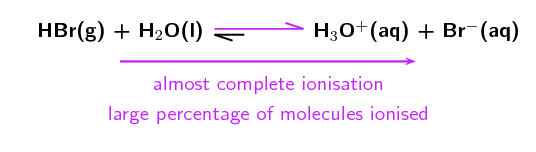The unequal double arrows in the reaction equation indicate that the equilibrium position favours the formation of ions. This is in contrast to the ionisation of a weak acid.

• Weak acids

Consider the ionisation of ethanoic acid ($$\text{CH}_{3}\text{COOH}$$):

$$\color{red}{\text{CH}_{3}{\text{COOH(aq)}}}$$ + $$\text{H}_{2}\text{O}(\text{ℓ})$$ $$\to$$ $$\text{H}_{3}\text{O}^{+}(\text{aq}) + \text{CH}_{3}\text{COO}^{-}(\text{aq})$$

Therefore, $$\color{red}{\text{K}_{\text{a}}} = \dfrac{\text{[H}_{3}{\text{O}}^{+}{\text{(aq)][CH}}_{3}{\text{COO}}^{-}{\text{(aq)]}}}{\color{red}{\text{[CH}_{3}{\text{COOH(aq)]}}}}$$

The value of $$\color{red}{\text{K}_{\text{a}}}$$ for this reaction is very low (approximately $$\text{1,7} \times \text{10}^{-\text{5}}$$), showing that only a few of the $$\text{CH}_{3}\text{COOH}$$ molecules ionise to form $$\text{H}_{3}\text{O}^{+}$$ (in water) and $$\text{CH}_{3}\text{COO}^{-}$$.

We can write the reaction with an unequal double arrow to show the position of the equilibrium, which does not favour the formation of ions: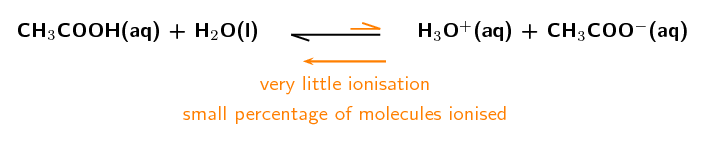Name Formula $$\text{K}_{\text{a}}$$ values Type Hydrobromic acid $$\text{HBr}$$ $$\text{1,0} \times \text{10}^{\text{9}}$$ strong acid Hydrochloric acid $$\text{HCl}$$ $$\text{1,3} \times \text{10}^{\text{6}}$$ strong acid Sulfuric acid $$\text{H}_{2}\text{SO}_{4}$$ First $$\text{H}^{+}$$: $$\text{1,0} \times \text{10}^{\text{3}}$$ Second $$\text{H}^{+}$$: $$\text{1,0} \times \text{10}^{-\text{2}}$$ strong acid Oxalic acid $$\text{H}_{2}\text{C}_{2}\text{O}_{4}$$ First $$\text{H}^{+}$$: $$\text{5,8} \times \text{10}^{-\text{2}}$$ Second $$\text{H}^{+}$$: $$\text{6,5} \times \text{10}^{-\text{5}}$$ weak acid Sulfurous acid $$\text{H}_{2}\text{SO}_{3}$$ First $$\text{H}^{+}$$: $$\text{1,4} \times \text{10}^{-\text{2}}$$ Second $$\text{H}^{+}$$: $$\text{6,3} \times \text{10}^{-\text{8}}$$ weak acid Hydrofluoric acid $$\text{HF}$$ $$\text{3,5} \times \text{10}^{-\text{4}}$$ weak acid Ethanoic acid $$\text{CH}_{3}\text{COOH}$$ $$\text{1,7} \times \text{10}^{-\text{5}}$$ weak acid

Table 9.5: $$\text{K}_{\text{a}}$$ values for the ionisation of some common acids. Note that sulfuric, oxalic and sulfurous acid can all lose $$\text{2}$$ $$\text{H}^{+}$$ ions (they are diprotic acids).

There are really only six strong inorganic acids, the rest are considered weak. These are:

$$\text{HClO}_{4}$$ (perchloric acid),

$$\text{HI}$$ (hydroiodic acid),

$$\text{HBr}$$ (hydrobromic acid),

$$\text{HCl}$$ (hydrochloric acid),

$$\text{H}_{2}\text{SO}_{4}$$ (sulfuric acid) and

$$\text{HNO}_{3}$$ (nitric acid).

#### Bases

The dissociation of bases is similar to that of acids in that we look at the $$\color{blue}{\text{K}_{\text{b}}}$$ values in a similar manner:

• Strong bases

For a strong base like $$\text{NaOH}$$:

$$\color{blue}{\text{NaOH(aq)}}$$ + $$\text{H}_{2}\text{O}(\text{ℓ})$$ $$\to$$ $$\text{Na}^{+}(\text{aq}) + \text{OH}^{-}(\text{aq}) + \text{H}_{2}\text{O}(\text{ℓ})$$

$$\color{blue}{\text{K}_{\text{b}}} = \dfrac{\text{[Na}^{+}{\text{(aq)][OH}}^{-}{\text{(aq)]}}}{\color{blue}{\text{[NaOH(aq)]}}}$$

The $$\color{blue}{\text{K}_{\text{b}}}$$ for $$\text{NaOH}$$ is very large ($$\text{NaOH}$$ is a strong base and almost completely dissociates) and shows that the equilibrium lies very far to the $$\text{OH}^{-}$$ side of the reaction. As a result we can write the equilibrium as: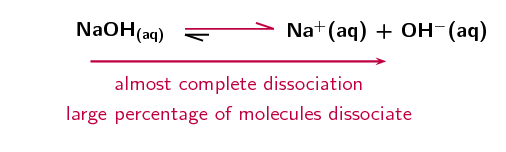• Weak bases

$$\color{blue}{\text{NH}_{3}{\text{(g)}}}$$ + $$\text{H}_{2}\text{O}(\text{ℓ})$$ $$\to$$ $$\text{NH}_{4}^{+}(\text{aq}) + \text{OH}^{-}(\text{aq})$$

$$\color{blue}{\text{K}_{\text{b}}} = \dfrac{\text{[NH}_{4}^{+}{\text{(aq)][OH}}^{-}{\text{(aq)]}}}{\color{blue}{\text{[NH}_{3}{\text{(g)]}}}}$$

The $$\color{blue}{\text{K}_{\text{b}}}$$ for $$\text{NH}_{3}$$ is approximately $$\text{1,8} \times \text{10}^{-\text{5}}$$ ($$\text{NH}_{3}$$ is a weak base) and shows that the equilibrium lies to the $$\text{NH}_{3}$$ side of the reaction. As a result we can write the equilibrium as: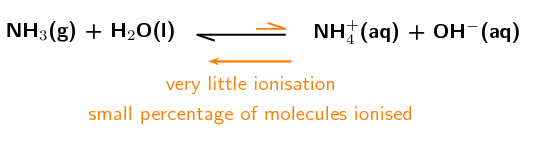## Worked example 6: Equilibrium constant calculations

Calculate the equilibrium constant for hydrochloric acid added to $$\text{1,38}$$ $$\text{dm^{3}}$$ of water:

$$\text{HCl}(\text{aq}) + \text{H}_{2}\text{O}(\text{ℓ})$$ $$\to$$ $$\text{H}_{3}\text{O}^{+}(\text{aq}) + \text{Cl}^{-}(\text{aq})$$

$$n(\text{HCl})$$ in solution = $$\text{0,005}$$ $$\text{mol}$$

$$n(\text{Cl}^{-})$$ in solution = $$\text{87,3}$$ $$\text{mol}$$

### Calculate the concentration of $$\text{HCl}$$ at equilibrium

$$c(\text{HCl}) = \dfrac{n}{V} = \dfrac{\text{0,005} {\text{ mol}}}{\text{1,38} {\text{ dm}}^{3}} = \text{0,0036} \text{ mol·dm^{-3}}$$

### Calculate the concentration of $$\text{Cl}^{-}$$ at equilibrium

$$c(\text{Cl}^{-}) = \dfrac{n}{V} = \dfrac{\text{87,3} {\text{ mol}}}{\text{1,38} {\text{ dm}}^{3}} = \text{63,3} \text{ mol·dm^{-3}}$$

### Calculate the concentration of $$\text{H}_{3}\text{O}^{+}$$ at equilibrium

There is a $$\text{1}$$:$$\text{1}$$ mole ratio between $$\text{H}_{3}\text{O}^{+}$$ and $$\text{Cl}^{-}$$. Therefore, if $$\text{87,3}$$ $$\text{mol}$$ of $$\text{Cl}^{-}$$ is present at equilibrium, $$\text{87,3}$$ $$\text{mol}$$ of $$\text{H}_{3}\text{O}^{+}$$ must be present as well.

$$c(\text{H}_{3}\text{O}^{+}) = \dfrac{n}{V} = \dfrac{\text{87,3} {\text{ mol}}}{\text{1,38} {\text{ dm}}^{3}} = \text{63,3} \text{ mol·dm^{-3}}$$

### Calculate $$\text{K}_{\text{a}}$$ (because $$\text{HCl}$$ is an acid)

$$\text{K}_{\text{a}} = \dfrac{\text{[H}_{3}{\text{O}}^{+}{\text{(aq)]}}^{1}{\text{[Cl}}^{-}{\text{(aq)]}}^{1}}{\text{[HCl]}^{1}}$$

$$\text{K}_{\text{a}} = \dfrac{\text{63,3} \times \text{63,3}}{\text{0,0036}}$$ = $$\text{1,11} \times \text{10}^{\text{6}}$$

In a balanced chemical equation such as:

$$2\text{C}$$ $$\to$$ $$\text{A} + \text{B}$$

$$\text{K}_{\text{a}}$$ = $$\dfrac{\text{[A]}^{1}\text{[B]}^{1}}{\text{[C]}^{2}}$$

However, we generally do not include the superscripts when the coefficients in the balanced equation are 1:

$$\text{K}_{\text{a}}$$ = $$\dfrac{\text{[A][B]}}{\text{[C]}^{2}}$$

Remember that $$\text{x}^{1}$$ = x.

## Worked example 7: Equilibrium constant calculations

The equilibrium constant ($$\text{K}_{\text{b}}$$) for the following reaction is $$\text{1,8} \times \text{10}^{-\text{5}}$$.

$$\text{NH}_{3}(\text{aq}) + \text{H}_{2}\text{O}(\text{ℓ})$$ $$\to$$ $$\text{NH}_{4}^{+}(\text{aq}) + \text{OH}^{-}(\text{aq})$$

Calculate the mass (at equilibrium) of $$\text{NH}_{3}$$ molecules dissolved in $$\text{4}$$ $$\text{dm^{3}}$$ of water if there is a $$\text{0,00175}$$ $$\text{mol·dm^{-3}}$$ concentration of hydroxide ions at equilibrium.

### What is the $$\text{K}_{\text{b}}$$ equation for this reaction

$$\text{K}_{\text{b}} = \dfrac{{\text{[NH}}_{4}^{+}{\text{(aq)]}}^{1}{\text{[OH}}^{-}{\text{(aq)]}}^{1}}{{\text{[NH}}_{3}{\text{(aq)]}}^{1}}$$

### Calculate the concentration of $$\text{NH}_{3}$$ at equilibrium

If there is a $$\text{0,00175}$$ $$\text{mol·dm^{-3}}$$ concentration of $$\text{OH}^{-}$$ ions at equilibrium there must be an equal concentration of $$\text{NH}_{4}^{+}$$ ions.

\begin{align*} \text{[NH}_{3}{\text{(aq)]}} & = \frac{{\text{[NH}}_{4}^{+}{\text{(aq)]}}^{1}{\text{[OH}}^{-}{\text{(aq)]}}^{1}}{{\text{K}}_{\text{b}}} \\ & = \dfrac{{\text{(}}\text{0,00175}{\text{)}}{\text{(}}\text{0,00175}{\text{)}}}{\text{1,8} \times \text{10}^{-\text{5}}} \\ & = \text{0,17}\text{ mol·dm$^{-3}$} \end{align*}

### Calculate the number of moles of $$\text{NH}_{3}$$ at equilibrium

$$c\text{ (mol·dm}^{-3}{\text{)}} = \dfrac{n\text{ (mol)}} {V\text{ (dm}^{3}{\text{)}}}$$, therefore:

$$n = c \times V = \text{0,17} \text{ mol·dm^{-3}} \times \text{4} \text{ dm^{3}} = \text{0,68} \text{ mol}$$

### Calculate the mass of $$\text{NH}_{3}$$ in solution at equilibrium

$$n\text{ (mol)} = \dfrac{m\text{ (g)}}{ M\text{ (g·mol}^{-1}{\text{)}}}$$. Therefore $$m = n \times M$$

$$M(\text{NH}_{3}) = \text{14,0} + (\text{3} \times \text{1,01}) = \text{17,03} \text{ g·mol^{-1}}$$

$$m = \text{0,68} \text{ mol} \times \text{17,03} \text{ g·mol^{-1}} = \text{11,6} \text{ g}$$

temp text

## Acids and bases

Textbook Exercise 9.3

State whether the acids and bases in the following balanced chemical equations are strong or weak and give a reason for your answer.

$$\text{HF}$$: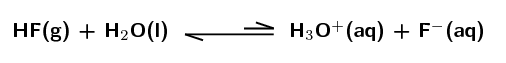Weak acid. The equilibrium lies far to the left meaning that only a small percentage of the hydrofluoric acid molecules donate a proton, ionisation is not favoured.

$$\text{KOH}$$ dissolved in water: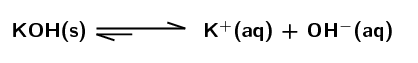Strong base. The equilibrium lies far to the right meaning that a large percentage of the potassium hydroxide molecules dissociate in water, dissociation is favoured.

$$\text{NH}_{3}$$: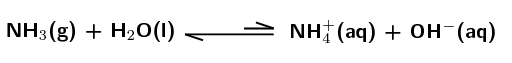Weak base. The equilibrium lies far to the left meaning that only a small percentage of the ammonia molecules accept a proton, ionisation is not favoured.

$$\text{HCl}$$: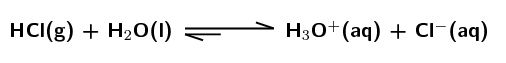Strong acid. The equilibrium lies far to the right meaning that a large percentage of the hydrochloric acid molecules donate a proton, ionisation is favoured.

State whether the acids and bases in the following questions are strong or weak and give a reason for your answer.

$$\text{CH}_{3}\text{COOH}$$ has a $$\text{K}_{\text{a}}$$ value of $$\text{1,7} \times \text{10}^{-\text{5}}$$.

Weak acid. $$\text{K}_{\text{a}}$$ is very small, meaning that only a small percentage of the ethanoic acid ($$\text{CH}_{3}\text{COOH}$$) molecules donate a proton, ionisation is not favoured.

$$\text{NH}_{3}$$ has a $$\text{K}_{\text{b}}$$ value of $$\text{1,8} \times \text{10}^{-\text{5}}$$.

Weak base. $$\text{K}_{\text{b}}$$ is very small, meaning that only a small percentage of the ammonia ($$\text{NH}_{3}$$) molecules accept a proton, ionisation is not favoured.

$$\text{HI}$$ has a $$\text{K}_{\text{a}}$$ value of $$\text{3,2} \times \text{10}^{\text{9}}$$.

Strong acid. $$\text{K}_{\text{a}}$$ is very large, meaning that almost all of the hydroiodic acid ($$\text{HI}$$) molecules donate a proton, ionisation is favoured.